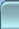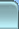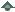< Blogs - MANIK.IN >
Sections________________ Parted Story LG GPRS Konqueror I ________________ ________________ ________________ Back to Blog Index

Web Log _

The Horrible C Exam

Manik Chand Patnaik
Fri Dec 29 22:30:36 2006

Today was the exam day for my students. I was particulary horrified for my students because of the questions they were asked to answer were rather ambiguous in nature.

The Starting

The starting question was Question number 1 with instructions to find the output of programs.

#include<stdio.h>
void main()
{
char *p="Hello !";
p="Hi hello";
*p='G';
printf("%s",p);
}

This question is very much dependent on the environment. Run it on Turbo C 3 and it gives the output  as the following

Gi hello

But  the very first declaration of *p would generate an illegal operation error (run time) if done with gcc.

#include<stdio.h>
int a=10;
void main()
{
int a=20;
{
int a=30;
printf("%d %d",a,::a);
}
}

The block of code on Turbo C 3 would give print 30 and 10 but on gcc it would give a compile time error.

#include<stdio.h>
int a=10;
void main()
{
int a=5,*p=&a;
printf("%d",++(*p));

}

The answer was 6 and very obvious.

#include<stdio.h>
int a=10;
void main()
{
int const * p=5;
printf("%d",++(*p));

}

This is error because once a memory location is declared read only it cannot be modified so the increment operation is not permitted.

void main()
{
char s[]="man";
int i;
for(i=0;s[i];i++)
printf("\n%c%c%c%c",s[i],*(s+i),*(i+s),i[s]);

}

This displays
mmmm
aaaa
nnnn